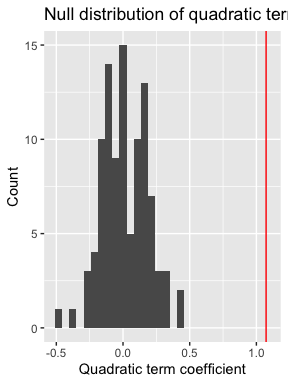# An introduction to the regressinator

The regressinator is a simulation system designed to make it easy to simulate different regression scenarios. By specifying the true population distribution of the predictors, and giving the exact relationship between predictors and response variables, we can simulate samples from the population easily. We can use these samples to explore questions like:

1. What do different diagnostic plots look like with different kinds of model misspecification?
2. How do regression estimators behave with different populations?
3. What happens to estimators when their assumptions are violated?

The regressinator is also a diagnostic package for regression models. Once a model is fit, it provides tools to extract various kind of residuals in convenient (tidy) form, to simulate from the sampling distribution of any desired model parameter, and to conduct simulation-based inference on the model fit, including visual inference with lineups.

The regressinator is intended for use in the classroom, as a tool for students to learn about regression in lab activities or homework assignments. Its flexibility makes it suitable for any regression course where students are expected to regularly use R.

## Population setup

Each simulation begins by specifying a population: the true population distribution the regression data comes from. Typically this is an infinite population of individuals whose covariates follow specified distributions. The outcome variable is defined to be a function of those covariates with some error. We use the population() function to define a population. For instance, this population has a simple linear relationship between two predictor variables, x1 and x2, and the response variable y:

library(regressinator)

linear_pop <- population(
x1 = predictor("rnorm", mean = 4, sd = 10),
x2 = predictor("runif", min = 0, max = 10),
y = response(
0.7 + 2.2 * x1 - 0.2 * x2, # relationship between X and Y
family = gaussian(),       # link function and response distribution
error_scale = 1.5          # errors are scaled by this amount
)
)

Notice how the predictors are defined using predictor(), each specifying its distribution (via the name of the R function used to simulate it, such as rnorm()) and any arguments to that distribution, such as mean or standard deviation. The response variable is defined by response(), whose first argument gives y as a function of the predictors. This expression can refer to the predictors by name, and will be used to simulate the response when sampling from the population. We can also specify the link and error scale. By default, the error distribution is Gaussian, and as the gaussian() family has errors with variance 1 by default, setting the error_scale to 1.5 means the errors have standard deviation 1.5.

More generally, population() defines a population according to the following relationship:

\begin{align*} Y &\sim \text{SomeDistribution} \\ g(\mathbb{E}[Y \mid X = x]) &= \mu(x) \\ \mu(x) &= \text{any function of } x. \end{align*}

The function $$\mu(x)$$ is the first argument to response(), and can be any arbitrary R expression. The distribution is given by the family argument, just like families of GLMs in glm(). If no family is provided, the default family is Gaussian, i.e. normally distributed errors, and the link function $$g$$ is the identity.

We can hence specify a population with binary outcomes and logistic link function:

logistic_pop <- population(
x1 = predictor("rnorm", mean = 0, sd = 10),
x2 = predictor("runif", min = 0, max = 10),
y = response(0.7 + 2.2 * x1 - 0.2 * x2,
)

This population specifies that

\begin{align*} Y &\sim \text{Bernoulli}\left(\text{logit}^{-1}(\mu(X))\right) \\ \mu(X) &= 0.7 + 2.2 X_1 - 0.2 X_2. \end{align*}

The regressinator supports the gaussian(), binomial(), and poisson() families from base R, and can draw response variables accordingly.

### Custom response distributions

It’s often useful to simulate data with non-standard response distributions, so we can investigate how estimators and diagnostics behave under different kinds of misspecification. The regressinator provides several custom response families to support this.

First, we can represent heteroskedasticity (unequal variance) in linear regression by using the error_scale argument to response(). This argument is evaluated in the sampled data frame’s environment, meaning it can be written in terms of the predictors. For example:

heteroskedastic_pop <- population(
x1 = predictor("rnorm", mean = 5, sd = 4),
x2 = predictor("runif", min = 0, max = 10),
y = response(
4 + 2 * x1 - 3 * x2, # relationship between X and Y
family = ols_with_error(rnorm), # distribution of the errors
error_scale = 0.5 + x2 / 10 # errors depend on x2
)
)

Next, the ols_with_error() family represents an identity link function with custom error distribution. For instance, in this population, the errors are $$t$$-distributed with 3 degrees of freedom:

heavy_tail_pop <- population(
x1 = predictor("rnorm", mean = 5, sd = 4),
x2 = predictor("runif", min = 0, max = 10),
y = response(
4 + 2 * x1 - 3 * x2, # relationship between X and Y
family = ols_with_error(rt, df = 3), # distribution of the errors
error_scale = 1.0 # errors are multiplied by this scale factor
)
)

Again, we can use error_scale to scale the error distribution to change its overall variance, and the error_scale can depend on the predictors.

Finally, use custom_family() to represent a completely arbitrary relationship, as defined by the response distribution and inverse link function. For instance, a zero-inflated Poisson family:

# 40% of draws have lambda = 0, the rest have lambda given by the inverse link
zeroinfpois <- function(ys) {
n <- length(ys)
rpois(n, lambda = ys * rbinom(n, 1, prob = 0.4))
}

pop <- population(
x1 = predictor("rnorm", mean = 2, sd = 2),
y = response(
0.7 + 0.8 * x1,
family = custom_family(zeroinfpois, exp)
)
)

Here y is sampled by evaluating zeroinfpois(exp(0.7 + 0.8 * x1)), and so 40% of the draws will be 0, and the rest will come from rpois(lambda = exp(0.7 + 0.8 * x1)). This approach could also be used to simulate contaminated error distributions, inject outliers, or produce other unusual error patterns.

### Categorical predictors

R supports many common probability distributions, so most common predictor variable distributions can be specified. But categorical predictor variables (factors) are very common in practice, and so the regressinator provides rfactor() for drawing factor variables:

# default is equally likely levels
rfactor(5, c("foo", "bar", "baz"))
#>  baz bar baz baz bar
#> Levels: foo bar baz

# but probabilities per level can be specified
rfactor(5, c("foo", "bar", "baz"), c(0.4, 0.3, 0.3))
#>  foo baz baz foo bar
#> Levels: foo bar baz

Then, using the by_level() helper, we can use factors in defining a regression model. For instance, here is a model with a different intercept per group:

intercepts <- c("foo" = 2, "bar" = 30, "baz" = 7)

factor_intercept_pop <- population(
group = predictor("rfactor",
levels = c("foo", "bar", "baz"),
prob = c(0.1, 0.6, 0.3)),
x = predictor("runif", min = 0, max = 10),
y = response(by_level(group, intercepts) + 0.3 * x,
error_scale = 1.5)
)

Or, similarly, a model with a different slope per group:

slopes <- c("foo" = 2, "bar" = 30, "baz" = 7)

factor_slope_pop <- population(
group = predictor("rfactor",
levels = c("foo", "bar", "baz"),
prob = c(0.1, 0.6, 0.3)),
x = predictor("runif", min = 0, max = 10),
y = response(7 + by_level(group, slopes) * x,
error_scale = 1.5)
)

## Sampling from the population

We can use sample_x() to get a sample from a population:

sample_x(linear_pop, n = 10)
#> Sample of 10 observations from
#> Population with variables:
#> x1: rnorm(list(mean = 4, sd = 10))
#> x2: runif(list(min = 0, max = 10))
#> y: gaussian(~0.7 + 2.2 * x1 - 0.2 * x2, error_scale = ~1.5)
#>
#> # A tibble: 10 × 2
#>         x1    x2
#>  *   <dbl> <dbl>
#>  1   0.394  3.32
#>  2   6.72   7.06
#>  3  13.5    9.21
#>  4 -24.4    8.99
#>  5   7.69   9.22
#>  6 -27.0    8.84
#>  7  13.3    3.52
#>  8  -9.43   8.46
#>  9  -0.700  6.22
#> 10   6.39   8.12

sample_y() then works on this sample and adds a y column, following the relationship specified in the population. Sampling X and Y is separated because often, in regression theory, we treat X as fixed – hence we want to conduct simulations where the same X data is used and we repeatedly draw new Y values according to the population relationship.

Using pipes, it’s easy to put together a simple simulation:

logistic_pop |>
sample_x(n = 10) |>
sample_y()
#> Sample of 10 observations from
#> Population with variables:
#> x1: rnorm(list(mean = 0, sd = 10))
#> x2: runif(list(min = 0, max = 10))
#> y: binomial(~0.7 + 2.2 * x1 - 0.2 * x2, error_scale = ~NULL, size = ~1L)
#>
#> # A tibble: 10 × 3
#>        x1    x2     y
#>     <dbl> <dbl> <int>
#>  1  -6.59 9.85      0
#>  2  -7.62 9.98      0
#>  3   1.42 3.59      1
#>  4   1.68 7.34      1
#>  5  10.7  8.90      1
#>  6  14.6  0.370     1
#>  7   6.47 3.68      1
#>  8  -4.12 4.75      0
#>  9 -11.7  2.46      0
#> 10 -10.4  6.79      0

The object this produces is a standard data frame (just with a few extra attributes), so it can be given to lm(), glm(), or any other standard modeling function that uses data frames.

## Fitting models

After we simulate a sample from a population, we can fit our chosen models to that sample. That model may simply use the predictors as-is, entering them into the design matrix and obtaining estimates through least square or maximum likelihood. But it is also common to represent the predictors differently in the model:

• Factor predictors are typically represented as several columns, for instance with one-hot encoding
• Predictors might be transformed, so the transformed version enters the model
• To fit a polynomial or spline, we might use several transformations of the predictor in the model (such as linear, squared, and cubed terms)

We call these transformed and recoded variables regressors. The coefficients of a model give the relationship between the regressors and the response, but the regressors may be transformed versions of the predictors. Many conventional diagnostics are based on the regressors, such as most residual plots, but as we will see below, others directly examine the relationship between the predictors and the response, however those predictors have been transformed and entered into the model.

## Producing diagnostics

Once we have a sample and fit a model, we can conduct diagnostics. Simple diagnostic plots can easily be made using broom::augment(), which generates a data frame with columns including the predictors, residuals, standardized residuals, fitted value, and other useful statistics for each observation. It also works for many common types of models; see the broom documentation for details.

For example, consider a simple dataset where the true relationship between x1 and y is not linear. We can quickly plot residuals versus fitted values:

library(broom)

nonlinear_pop <- population(
x1 = predictor("runif", min = 1, max = 8),
x2 = predictor("runif", min = 0, max = 10),
y = response(0.7 + x1**2 - x2, family = gaussian(),
error_scale = 4.0)
)

nonlinear_data <- nonlinear_pop |>
sample_x(n = 100) |>
sample_y()

nonlinear_fit <- lm(y ~ x1 + x2, data = nonlinear_data)

# to see the kind of information we get from an augmented fit
augment(nonlinear_fit)
#> # A tibble: 100 × 9
#>        y    x1    x2 .fitted .resid   .hat .sigma  .cooksd .std.resid
#>    <dbl> <dbl> <dbl>   <dbl>  <dbl>  <dbl>  <dbl>    <dbl>      <dbl>
#>  1  9.51  2.34 0.893    5.13   4.38 0.0417   5.51 0.00957       0.813
#>  2  5.61  3.08 5.45     6.81  -1.20 0.0150   5.53 0.000245     -0.219
#>  3 -1.70  1.19 8.32   -13.1   11.4  0.0504   5.40 0.0805        2.13
#>  4 19.0   5.66 8.78    26.3   -7.31 0.0299   5.48 0.0187       -1.35
#>  5 19.6   4.69 4.70    22.0   -2.38 0.0104   5.53 0.000663     -0.435
#>  6 58.5   7.72 4.59    49.2    9.26 0.0402   5.45 0.0412        1.72
#>  7 42.0   6.83 2.27    43.8   -1.80 0.0351   5.53 0.00135      -0.333
#>  8  3.71  3.52 5.46    10.8   -7.09 0.0123   5.49 0.00697      -1.30
#>  9 10.9   4.06 5.61    15.4   -4.55 0.0107   5.51 0.00248      -0.831
#> 10  4.07  3.38 3.56    11.6   -7.49 0.0155   5.48 0.00987      -1.37
#> # ℹ 90 more rows

library(ggplot2)

ggplot(augment(nonlinear_fit),
aes(x = .fitted, y = .resid)) +
geom_point() +
labs(x = "Fitted value", y = "Residual")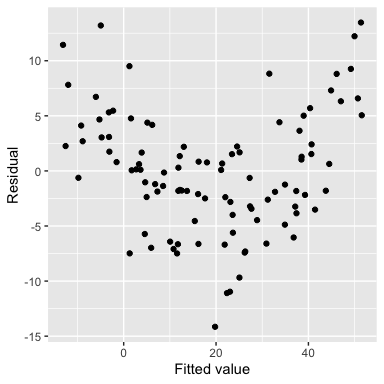The regressinator builds on these tools to support additional diagnostics. For example, augment_longer() is like broom::augment(), but produces one row per regressor per observation, essentially putting the data in “long” format. This makes it easy to facet the data to plot residuals against each regressor:

ggplot(augment_longer(nonlinear_fit),
aes(x = .predictor_value, y = .resid)) +
geom_point() +
facet_wrap(vars(.predictor_name), scales = "free") +
labs(x = "Predictor value", y = "Residual")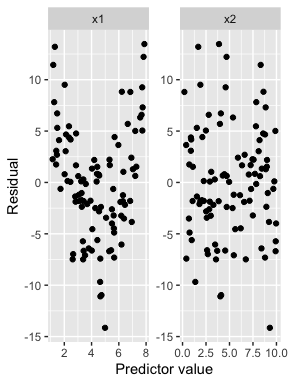Partial residuals are an extension of ordinary residuals that are defined in terms of the original predictors, not the regressors; we can think of a partial residual plot for a particular predictor as showing the relationship between that predictor and the response, after “adjusting for” the other predictors according to our fitted model. The partial_residuals() function calculates partial residuals in tidy format, and its documentation provides detailed references on the use and interpretation of partial residuals.

Here the black line gives the fitted predictor effects (i.e. the model estimate of the relationship), while the blue line smooths the partial residuals. We can see that for x1, the blue line deviates systematically from the black line in a quadratic shape:

ggplot(partial_residuals(nonlinear_fit),
aes(x = .predictor_value, y = .partial_resid)) +
geom_point() + # partial residuals
geom_smooth(se = FALSE) + # smoothed residuals
geom_line(aes(x = .predictor_value, y = .predictor_effect)) + # effects
facet_wrap(vars(.predictor_name), scales = "free") +
labs(x = "Predictor value", y = "Partial residual")
#> geom_smooth() using method = 'loess' and formula = 'y ~ x'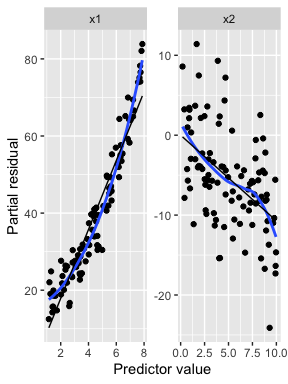If we fit a quadratic model for x1, the partial residuals reflect this. The black line for the effect of x1 is now quadratic, and the partial residuals closely follow it:

quadratic_fit <- lm(y ~ poly(x1, 2) + x2, data = nonlinear_data)

aes(x = .predictor_value, y = .partial_resid)) +
geom_point() + # partial residuals
geom_smooth(se = FALSE) + # smoothed residuals
geom_line(aes(x = .predictor_value, y = .predictor_effect)) + # effects
facet_wrap(vars(.predictor_name), scales = "free") +
labs(x = "Predictor value", y = "Partial residual")
#> geom_smooth() using method = 'loess' and formula = 'y ~ x'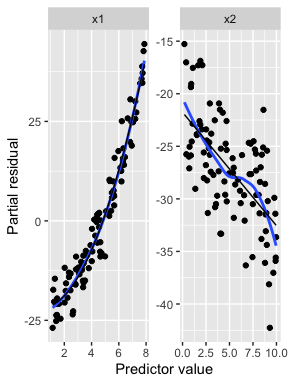These diagnostic functions work for linear models and also GLMs fit by glm(). See vignette("linear-regression-diagnostics") and vignette("logistic-regression-diagnostics") for further diagnostic examples.

## Visual inference

It can be difficult to tell whether a particular pattern in model diagnostics signifies a real problem or just an unlucky pattern. To help make this judgment, the regressinator provides the model_lineup() function. It takes a model fit and does the following:

1. Get the diagnostics from that model, using broom::augment() by default.
2. Simulate 19 additional datasets using the model, using the standard assumptions for that model. (For instance, for linear regression, errors are drawn from a normal distribution.) In each dataset, the same X values are used, and only new Y values are drawn. Each dataset is the same size.
3. For each of those datasets, fit the same model and calculate the diagnostics.
4. Put the diagnostics for all 20 fits into one data frame with a .sample column indicating which fit each diagnostic came from.
5. Print out a message indicating how to tell which of the .sample values comes from the original model.

This helps us compare how diagnostic plots will look when assumptions hold (by looking at the 19 simulated datasets) to how the diagnostic plots of our real model look. This visual inference approach is taken from the nullabor package.

For example, consider the same dataset where the true relationship is not linear, but we use a linear fit. We can quickly plot residuals versus fitted values:

model_lineup(nonlinear_fit) |>
ggplot(aes(x = .fitted, y = .resid)) +
geom_point() +
facet_wrap(vars(.sample)) +
labs(x = "Fitted value", y = "Residual")
#> decrypt("nsW7 Ykjk l3 gCPljlC3 KT")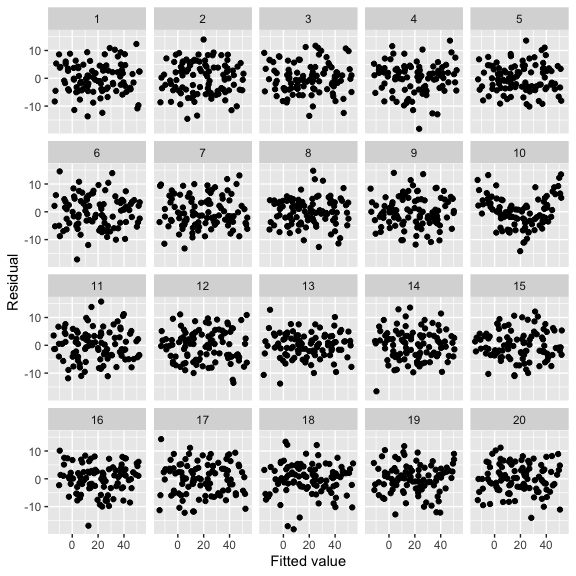The user can examine the 20 plots and guess which one represents the model fit to the original data, and which are generated assuming the fitted model is correct. The decrypt() code provided will print out a message indicating which of the plots is the real data; in this case, it’s the plot with the clear quadratic trend in the residuals.

This approach can be used to explore different kind of misspecification. For example, using ols_with_error() we can test whether we can spot non-normal residual distributions in residual Q-Q plots:

heavy_tail_pop <- population(
x1 = predictor("rnorm", mean = 5, sd = 4),
x2 = predictor("runif", min = 0, max = 10),
y = response(
4 + 2 * x1 - 3 * x2,
family = ols_with_error(rt, df = 3),
error_scale = 1.0
)
)

heavy_tail_sample <- heavy_tail_pop |>
sample_x(n = 100) |>
sample_y()

fit <- lm(y ~ x1 + x2, data = heavy_tail_sample)

model_lineup(fit) |>
ggplot(aes(sample = .resid)) +
geom_qq() +
geom_qq_line() +
facet_wrap(vars(.sample)) +
labs(x = "Theoretical quantiles", y = "Observed quantiles")
#> decrypt("nsW7 Ykjk l3 gCPljlC3 KK")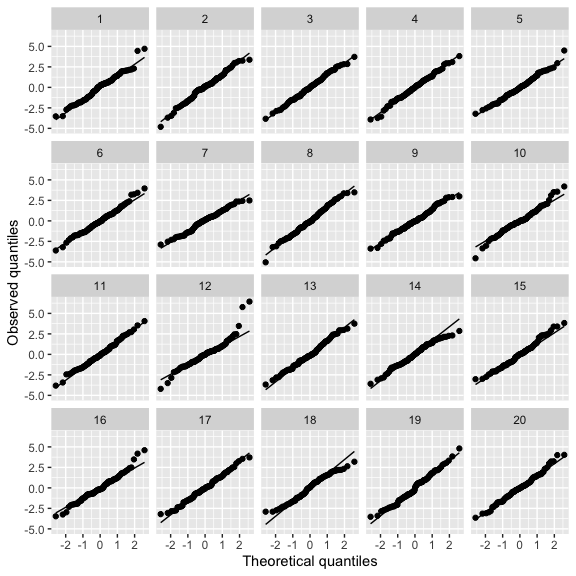Here we have Q-Q plots of residuals for a linear regression where the true relationship is linear, but the error distribution is $$t_3$$. This is a good way to see if we can spot the heavy-tailed distribution from among the 19 other Q-Q plots that simulate normally distributed errors.

## Sampling distributions

The sampling_distribution() function allows you to explore the sampling distribution of statistics and estimates from models fit to a population. Given a dataset drawn from a population() and a model fit to that data, it will:

1. Draw repeated samples from the population. These can be with the predictors held fixed, only resampling the response, or can be new samples of predictors and response jointly. Each sample is the same size as the original dataset.
2. For each sample, refit the model to that sample.
3. For each model fit, apply a function to get features of the model fit.
4. Combine all the results into a single data frame.

By default, the function applied to each fit is broom::tidy(), which can take many types of model fits and produce a data frame of the model coefficients and their standard errors.

We can hence explore the sampling distribution of estimates fit to samples from a population:

d <- linear_pop |>
sample_x(n = 30) |>
sample_y()

fit <- lm(y ~ x1 + x2, data = d)
tidy(fit)
#> # A tibble: 3 × 5
#>   term        estimate std.error statistic  p.value
#>   <chr>          <dbl>     <dbl>     <dbl>    <dbl>
#> 1 (Intercept)   0.0449    0.530     0.0847 9.33e- 1
#> 2 x1            2.19      0.0266   82.5    5.39e-34
#> 3 x2           -0.106     0.0937   -1.13   2.69e- 1

sampling_distribution(fit, d, nsim = 4)
#> # A tibble: 15 × 6
#>    term        estimate std.error statistic  p.value .sample
#>    <chr>          <dbl>     <dbl>     <dbl>    <dbl>   <dbl>
#>  1 (Intercept)   0.0449    0.530     0.0847 9.33e- 1       0
#>  2 x1            2.19      0.0266   82.5    5.39e-34       0
#>  3 x2           -0.106     0.0937   -1.13   2.69e- 1       0
#>  4 (Intercept)   0.505     0.480     1.05   3.03e- 1       1
#>  5 x1            2.19      0.0241   90.9    4.07e-35       1
#>  6 x2           -0.149     0.0850   -1.75   9.12e- 2       1
#>  7 (Intercept)   1.07      0.564     1.91   6.74e- 2       2
#>  8 x1            2.18      0.0283   77.1    3.38e-33       2
#>  9 x2           -0.181     0.0997   -1.82   8.03e- 2       2
#> 10 (Intercept)   0.424     0.528     0.802  4.29e- 1       3
#> 11 x1            2.19      0.0265   82.6    5.25e-34       3
#> 12 x2           -0.0799    0.0934   -0.855  4.00e- 1       3
#> 13 (Intercept)   0.298     0.460     0.649  5.22e- 1       4
#> 14 x1            2.21      0.0231   95.6    1.03e-35       4
#> 15 x2           -0.133     0.0813   -1.64   1.12e- 1       4

Because the output is a tidy data frame, it’s easy to visualize the full sampling distributions:

samples <- sampling_distribution(fit, d, nsim = 1000)

samples |>
ggplot(aes(x = estimate)) +
geom_histogram() +
facet_wrap(vars(term), scales = "free") +
labs(x = "Estimate", y = "Frequency")
#> stat_bin() using bins = 30. Pick better value with binwidth.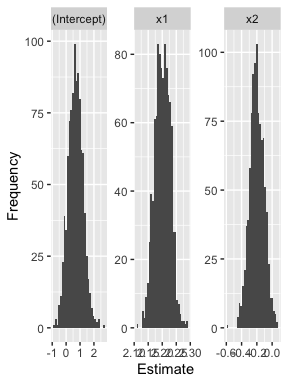Or to get the mean and standard deviation of the sampling distribution:

library(dplyr)

samples |>
group_by(term) |>
summarize(mean = mean(estimate),
sd = sd(estimate))
#> # A tibble: 3 × 3
#>   term          mean     sd
#>   <chr>        <dbl>  <dbl>
#> 1 (Intercept)  0.677 0.525
#> 2 x1           2.20  0.0273
#> 3 x2          -0.201 0.0942

We could use this feature to explore how sampling distributions change as we change the sample size, change the complexity of the model, or introduce various types of model misspecification.

## Parametric bootstrapping

The parametric_boot_distribution() function allows you to explore the distribution of statistics and estimates from models fit to data sampled from the model fit. Given a fitted model, it will:

1. Draw repeated samples from the model. The predictors are held fixed and the model is used to sample new response values, following the response and error distributions assumed by the model.
2. For each sample, refit a model to that sample. This could be the same model used to simulate the sample, for instance to explore diagnostics when the model is true, or an alternative model.
3. For each model fit, apply a function to get features of that model fit.
4. Combined all the results in to a single data frame.

As with sampling_distribution(), the default function applied to each fit is broom::tidy().

This function is used by model_lineup() to generate the “null” diagnostics. It could also be used for hypothesis testing. For example, let’s consider the nonlinear example set up above. The data nonlinear_data comes from a population where the true relationship between y and x1 is quadratic, but nonlinear_fit is a model fit with only a linear term. If we did not know a quadratic term was necessary and wanted to conduct a test, we could use an F test:

quadratic_fit <- lm(y ~ x1 + I(x1^2) + x2, data = nonlinear_data)

#> Analysis of Variance Table
#>
#> Model 1: y ~ x1 + x2
#> Model 2: y ~ x1 + I(x1^2) + x2
#>   Res.Df    RSS Df Sum of Sq      F    Pr(>F)
#> 1     97 2939.9
#> 2     96 1496.8  1      1443 92.549 9.735e-16 ***
#> ---
#> Signif. codes:  0 '***' 0.001 '**' 0.01 '*' 0.05 '.' 0.1 ' ' 1

This suggests the quadratic term is useful, as we would expect when the true relationship is quadratic and we have an adequate sample size. But if for some reason we do not want to use an F test, or if we are teaching simulation-based inference, we could instead use the parametric bootstrap. Under the null hypothesis, there is no quadratic relationship, so we simulate the null distribution of coefficient values under this null by simulating from nonlinear_fit and refitting quadratic_fit:

quadratic_coefs <- parametric_boot_distribution(nonlinear_fit, quadratic_fit) |>
filter(term == "I(x1^2)")

#> stat_bin() using bins = 30. Pick better value with binwidth.